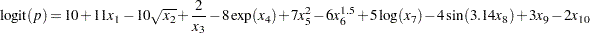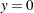## Example 68.1 Scoring with PROC PLM

Logistic regression with model selection is often used to extract useful information and build interpretable models for classification problems with many variables. This example demonstrates how you can use PROC LOGISTIC to build a spline model on a simulated data set and how you can later use the fitted model to classify new observations.

The following DATA step creates a data set named SimuData, which contains 5,000 observations andcontinuous variables:

```%let nObs   = 5000;
%let nVars  = 100;
data SimuData;
array x{&nVars};
do obsNum=1 to &nObs;
do j=1 to &nVars;
x{j}=ranuni(1);
end;

linp =  10 + 11*x1 - 10*sqrt(x2) + 2/x3 - 8*exp(x4) + 7*x5*x5
- 6*x6**1.5 + 5*log(x7) - 4*sin(3.14*x8) + 3*x9 - 2*x10;
TrueProb = 1/(1+exp(-linp));

if ranuni(1) < TrueProb then y=1;
else y=0;
output;
end;
run;
```

The response is binary based on the inversely transformed logit values. The true logit is a function of only 10 of the 100 variables, including nonlinear transformations of seven variables, as follows:Now suppose the true model is not known. With some exploratory data analysis, you determine that the dependency of the logit on some variables is nonlinear. Therefore, you decide to use splines to model this nonlinear dependence. Also, you want to use stepwise regression to remove unimportant variable transformations. The following statements perform the task:

```proc logistic data=SimuData;
effect splines = spline(x1-x&nVars/separate);
model y = splines/selection=stepwise;
store sasuser.SimuModel;
run;
```

By default, PROC LOGISTIC models the probability that. The EFFECT statement requests an effect named splines constructed by all predictors in the data. The SEPARATE option specifies that the spline basis for each variable be treated as a separate set so that model selection applies to each individual set. The SELECTION=STEPWISE specifies the stepwise regression as the model selection technique. The STORE statement requests that the fitted model be saved to an item store sasuser.SimuModel. See Working with Item Stores for an example with more details about working with item stores.

The spline effect for each predictor produces seven columns in the design matrix, making stepwise regression computationally intensive. For example, a typical Pentium 4 workstation takes around ten minutes to run the preceding statements. Real data sets for classification can be much larger. See examples at UCI Machine Learning Repository (Asuncion and Newman 2007). If new observations about which you want to make predictions are available at model fitting time, you can add the SCORE statement in the LOGISTIC procedure. However, if observations to predict become available after fitting the model, you must use the LOGISTIC procedure to refit the model to make predictions for new observations. With PROC PLM, you do not have to repeat the intimidating model-fitting processes multiple times. You can use the SCORE statement in the PLM procedure to score new observations based on the item store sasuser.SimuModel that was created during the initial model building. For example, to compute the probability offor one new observation with all predictor values equal to 0.15 in the data set test, you can use the following statements:

```data test;
array x{&nVars};
do j=1 to &nVars;
x{j}=0.15;
end;
drop j;
output;
run;
```
```proc plm source=sasuser.SimuModel;
score data=test out=testout predicted / ilink;
run;
```

The ILINK option in the SCORE statement requestes that predicted values be inversely transformed to the response scale. In this case, it is the predicted probability of. Output 68.1.1 shows the predicted probability for the new observation.

Output 68.1.1 Predicted Probability for One New Observation
Obs Predicted
1 0.56649